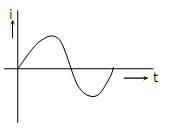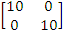# Given two continuous time signals x(t) =e-t and y (t)=e-2t which exist for t>0,the convolution.z(t)=x(t)*y(t) is

1.  e-t-e-2t

2.  e-3t

3.   e+t

4.  e-t+e-2t

4

e-t-e-2t

Explanation :
No Explanation available for this question

# A single phase air core transformer, fed from a rated sinusoidal supply, is operating at no load. The steady state magnetizing current drawn by the transformer from the supply will have the waveform

1.

2.

3.

4.

4Explanation :
No Explanation available for this question

# A negative sequence relay is commonly used to protect

1.  an alternator

2.  an transformer

3.  a transmission line

4.  a bus bar

4

an alternator

Explanation :
No Explanation available for this question

# For enhancing the power transmission in along EHV transmission line,the most preferred method is to connect a

1.  Series inductive compensator in the line

2.  Shunt inductive compensator at the receiving end

3.  Series capacitive compensator in the line

4.  Shunt capacitive compensator at the sending end

4

Series capacitive compensator in the line

Explanation :
No Explanation available for this question

# An open loop system represented by the transfer function G(s) = (s -1)/(s + 2)(s + 3) is

1.  Stable and of the minimum phase type

2.  Stable and of the non - minimum phase type

3.  Unstable and of the minimum phase type

4.  Unstable and of non-minimum phase type

4

Stable and of the non - minimum phase type

Explanation :
No Explanation available for this question

# The bridge circuit shown in the figure below is used for the measurement of an unknown element ZX. The bridge circuit is best suited when ZX is a

1.  low resistance

2.  low Q inductor

3.  high resistance

4.  loss capacitor

4

high resistance

Explanation :
No Explanation available for this question

# A dual trace oscilloscope is set to operate in the ALTernate mode. The control input of the multiplexer used in the y-circuit is fed with a signal having a frequency equal to

1.  the highest frequency that the multiplexer can operate properly

2.  twice the frequency of the time base (sweep) oscillator

3.  the frequency of the time base (sweep) oscillator

4.  half the frequency of the time base (sweep) oscillator

4

the frequency of the time base (sweep) oscillator

Explanation :
No Explanation available for this question

# The output Y of the logic circuit given below is

1.  1

2.  0

3.  X

4.

4

1

Explanation :
No Explanation available for this question

# Circuit turn-off time of an SCR is defined as the time

1.  taken by the SCR turn of

2.  required for the SCR current to become zero

3.  for which the SCR is reverse biased by the commutation circuit

4.  for which the SCR is reverse biased to reduce its current below the holding current

4

for which the SCR is reverse biased by the commutation circuit

Explanation :
No Explanation available for this question

# Solution of the variables x1 and x2 for the following equations is to be obtained by employing the Newton-Raphson iterative method. equation (i) 10x2 sin X1 - 0.8 = 0 equation (ii) 10X22 -10x2 Cos X1 - 0.6 = 0        Assuming the initial valued x1 = 0.0 and x2 = 1.0, the jacobian matrix is

1.

2.

3.

4.

4